Sie sind hier: ICP » R. Hilfer » Publikationen

1 Introduction

[page 89, §1]
[89.1.1] A large number of problems in theoretical physics, including Schrödingers, Maxwell and Newtons equations, can be formulated as initial value problems for dynamical evolution equations of the form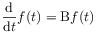(1)

where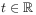denotes time and B is an operator on a Banach space. [89.1.2] Depending on the initial data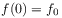describing the state or observable of the system at time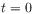the problem is to find the state or observable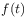of the system at later times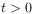. a (This is a footnote:) a In classical mechanics the states are points in phase space, the observables are functions on phase space, and the operator B is specified by a vector field and Poisson brackets. In quantum mechanics (with finitely many degrees of freedom) the states correspond to rays in a Hilbert space, the observables to operators on this space, and the operator B to the Hamiltonian. In field theories the states are normalized positive functionals on an algebra of operators or observables, and then B becomes a derivation on the algebra of observables. The equations (1) need not be first order in time. An example is the initial-value problem for the wave equation for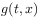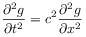in one dimension. It can be recast into the form of eq. (1) by introducing a second variable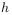and defining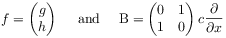[89.2.1] Many authors, mostly driven by the needs of applied problems, have considered generalizations of equation (1) of the form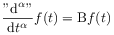(2)

in which the first order time derivative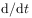is replaced with a certain fractional time derivative ‘‘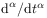’’ of order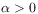(see e.g.   and the Chapters IV–VIII  in this book). [89.2.2] A number of fundamental questions are raised by such a replacement. [89.2.3] In order to appreciate these it is useful to recall that the appearance ofin eq. (1) reflects not only a basic symmetry of nature but also the basic principle of locality. [89.2.4] Of course, the symmetry in question is time translation invariance. [89.2.5] Remember that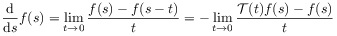(3)

[page 90, §0]    identifies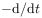as the infinitesimal generator of time translations b (This is a footnote:) b A simple translation with unit "speed" reflects the idea of time "flowing" uniformly with constant velocity. This idea is embodied in measuring time by comparison with periodic processes (clocks). A competing idea, related to the flow of time represented by eq. (5), is to measure time by comparison with nonperiodic clocks such as decay or aging processes. defined by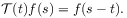(4)

[90.0.1] Equation (2) abandons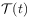as the general time evolution, and this raises the question what replaces eq. (4), and how a fractional derivative can arise as the generator of a physical time evolution. [90.0.2] Most workers in fractional calculus have avoided these questions, and my purpose in this chapter is to review and discuss an answer provided recently in [6, 7, 8, 9, 10, 11].

[90.1.1] Derivatives of fractional order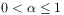were found to emerge quite generally as the infinitesimal generators of coarse grained macroscopic time evolutions given by [6, 7, 8, 9, 10, 11]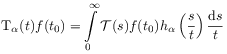(5)

where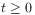and. [90.1.2] Explicit expressions for the kernels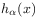for allare given in eq. (69) below. [90.1.3] It is the main objective of this chapter to show that (in a certain sense) all macroscopic time evolutions have the form of eq. (5), and that fractional time derivatives are their infinitesimal generators.

[90.2.1] Given the great difference between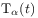in eq. (5) and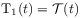in eq. (4) it becomes clear that basic issues, such as irreversibility, translation symmetry, or the meaning of stationarity are inevitably involved when proposing fractional dynamics. [90.2.2] Let me therefore advance the basic postulate that all time evolutions of physical systems are irreversible. [90.2.3] Obviously this law of irreversibility must be considered to be an empirical law of nature equal in rank to the law of energy conservation. [90.2.4] Reversible behaviour is an idealization. [90.2.5] Its validity or applicability in physical experiments depends on the degree to which the system can be isolated (or decoupled) from its past history and its environment. [90.2.6] According to this view the irreversible flow of time is more fundamental than the time reversal symmetry of Newtons or other equations. [90.2.7] My starting point is therefore that for a general time evolution operator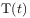the evolution parameter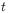is not a time instant (which could be positive or negative), but a duration, which cannot be negative.

[90.3.1] An immediate consequence of the postulated law of irreversibility is that the classical irreversibility problem of theoretical physics becomes reversed.

[page 91, §0]    [91.0.1] Now the theoretical task is not to explain how irreversibility arises from reversible evolution equations, but how seemingly reversible equations arise as idealizations from an underlying irreversible time evolution. [91.0.2] A possible explanation is provided by the present theory based on eq. (5). [91.0.3] It turns out that the case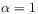in eq. (5) is of predominant mathematical and physical importance, because it is in a quantifiable sense a strong universal attractor. [91.0.4] In this case the kernel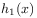becomes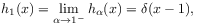(6)

and the time evolutionin (5) reduces to a simple translation as in eq.(4). [91.0.5]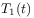withis a representation of the time semigroup (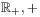). [91.0.6] It can be extended to one of the full group (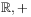). [91.0.7] This is not possible for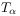with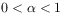. [91.0.8] The physical interpretation of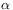is seen from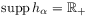for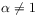and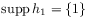for. [91.0.9] Hence the parameterclassifies and quantifies the influence of the past history. [91.0.10] Small values ofcorrespond to a strong influence of the past history. [91.0.11] Forthe influence of the past history is minimal in the sense that it enters only through the present state.

[91.1.1] The basic result in eq. (5) was given in  and subsequently rationalized within ergodic theory by investigating the recurrence properties of induced automorphisms on subsets of measure zero [9, 10, 11]. [91.1.2] In these investigations the existence of a recurrent subset of measure zero had to be assumed. [91.1.3] Such an assumption becomes plausible from observations in low dimensional chaotic systems (see e.g. [25, 26] and Chapter V). [91.1.4] A rigorous proof for any given dynamical system, however, appears difficult, and it is therefore of interest to rederive the emergence offrom a different, and more general, approach.